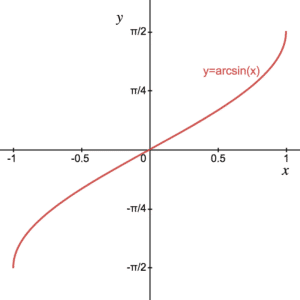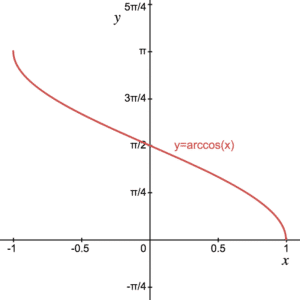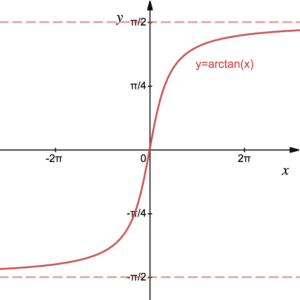# Inverse Trigonometric Functions: arcsin, arccos and arctan

The inverse trigonometric functions are $\arcsin(x)$, $\arccos(x)$ and $\arctan(x)$. These functions perform the reverse operations to the original trigonometric functions $\sin(x)$, $\cos(x)$ and $\tan(x)$ respectively. Recall that a function is invertible if it is one-to-one. Click here to revise inverse functions. Hence, before we can sketch the graphs of the inverse trigonometric functions, we must choose a domain for them for which they are one-to-one. Note that the original trigonometric functions work on angles and so each of the inverse trigonometric functions will return an angle. We use radians for all angles in the following – see more on radians. Also note that we use $\arcsin(x)$ instead of $\sin^{-1}(x)$, for example, as this can be confused with $\text{cosec}(x)$, the reciprocal trigonometric function

## Inverse Trigonometric Function: arcsin(x)Since $\sin(x)$ is periodic, there are infinitely many regions for which it is one-to-one. We choose the default domain to be $\frac{-\pi}{2}\leq x\leq \frac{\pi}{2}$. The range of $\sin(x)$ is $-1\leq\sin(x)\leq 1$.  It follows that the domain of $\text{arcsin}(x)$ is $-1\leq x\leq 1$ and the range is $\frac{-\pi}{2}\leq \text{arcsin}(x)\leq \frac{\pi}{2}$. The graphs of $\sin(x)$ and $\text{arcsin}(x)$ are reflections of one another in the line $y=x$.

## Inverse Trigonometric Function: arccos(x)Since $\cos(x)$ is periodic, there are infinitely many regions for which it is one-to-one. We choose the default domain to be $0\leq x\leq \pi$. The range of $\cos(x)$ is $-1\leq\cos(x)\leq 1$.  It follows that the domain of $\text{arccos}(x)$ is $-1\leq x\leq 1$ and the range is $0\leq \text{arcsin}(x)\leq \pi$. The graphs of $\cos(x)$ and $\text{arccos}(x)$ are reflections of one another in the line $y=x$.

## Inverse Trigonometric Function: arctan(x)Since $\tan(x)$ is periodic, there are infinitely many regions for which it is one-to-one. We choose the default domain to be $\frac{-\pi}{2}< x< \frac{\pi}{2}$. The range of $\tan(x)$ is all of ${\mathbb R}$.  It follows that the domain of $\text{arctan}(x)$ is $x\in{\mathbb R}$ and the range is $\frac{-\pi}{2}< \text{arctan}(x)< \frac{\pi}{2}$. The graphs of $\tan(x)$ and $\text{arctan}(x)$ are reflections of one another in the line $y=x$.

## Examples

Find the values (in radians) of the following without using a calculator:

1. $\text{arcsin}(1)$
2. $\text{arccos}(\cos(\pi/7))$
3. $\sin(\text{arctan}(1))$

Sketch the graph of $y=2\text{arccos}(-x)$, stating its domain and range.

Given that $\theta=\text{arccos}(x)$ where $\theta$ is an acute angle, find an expression for $\sin(\theta)$ in terms of $x$.